Question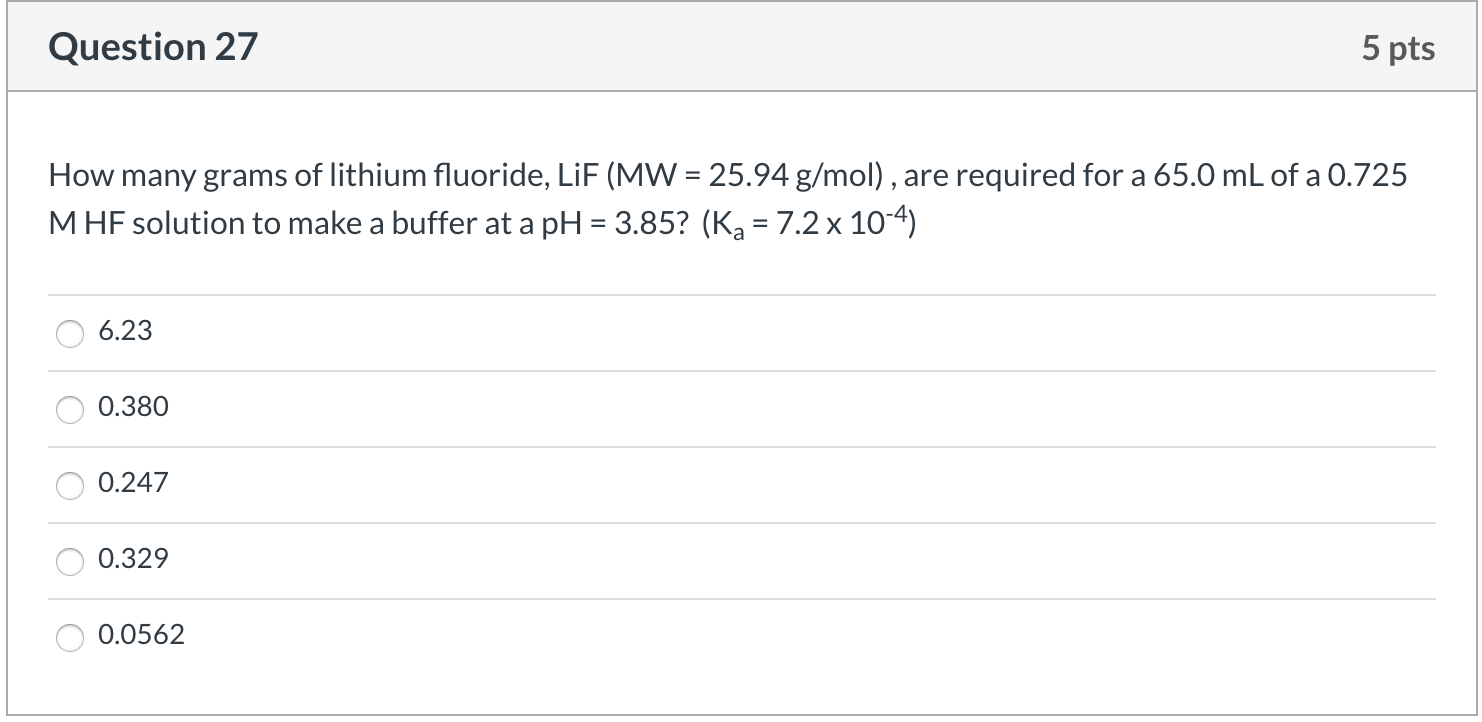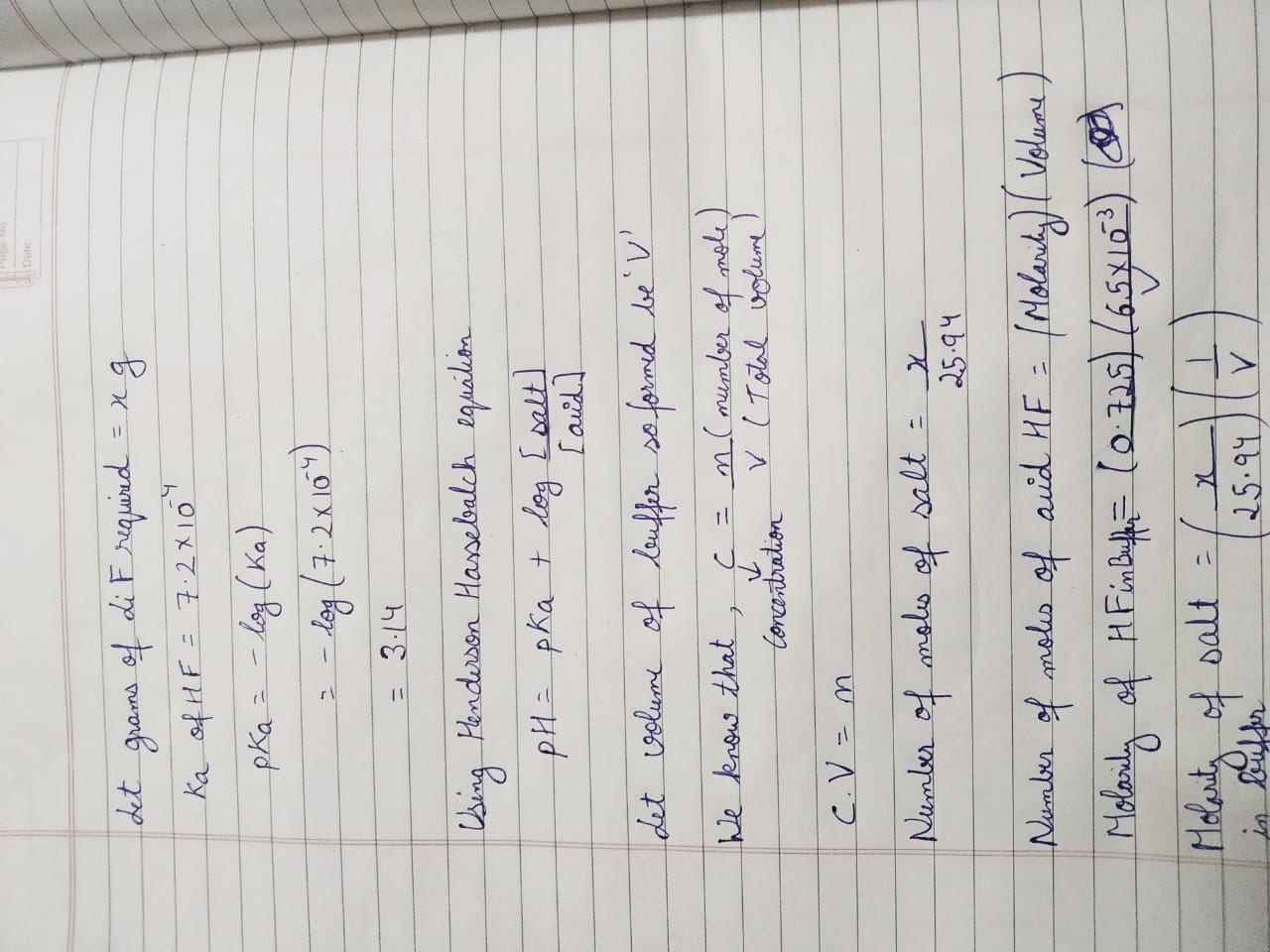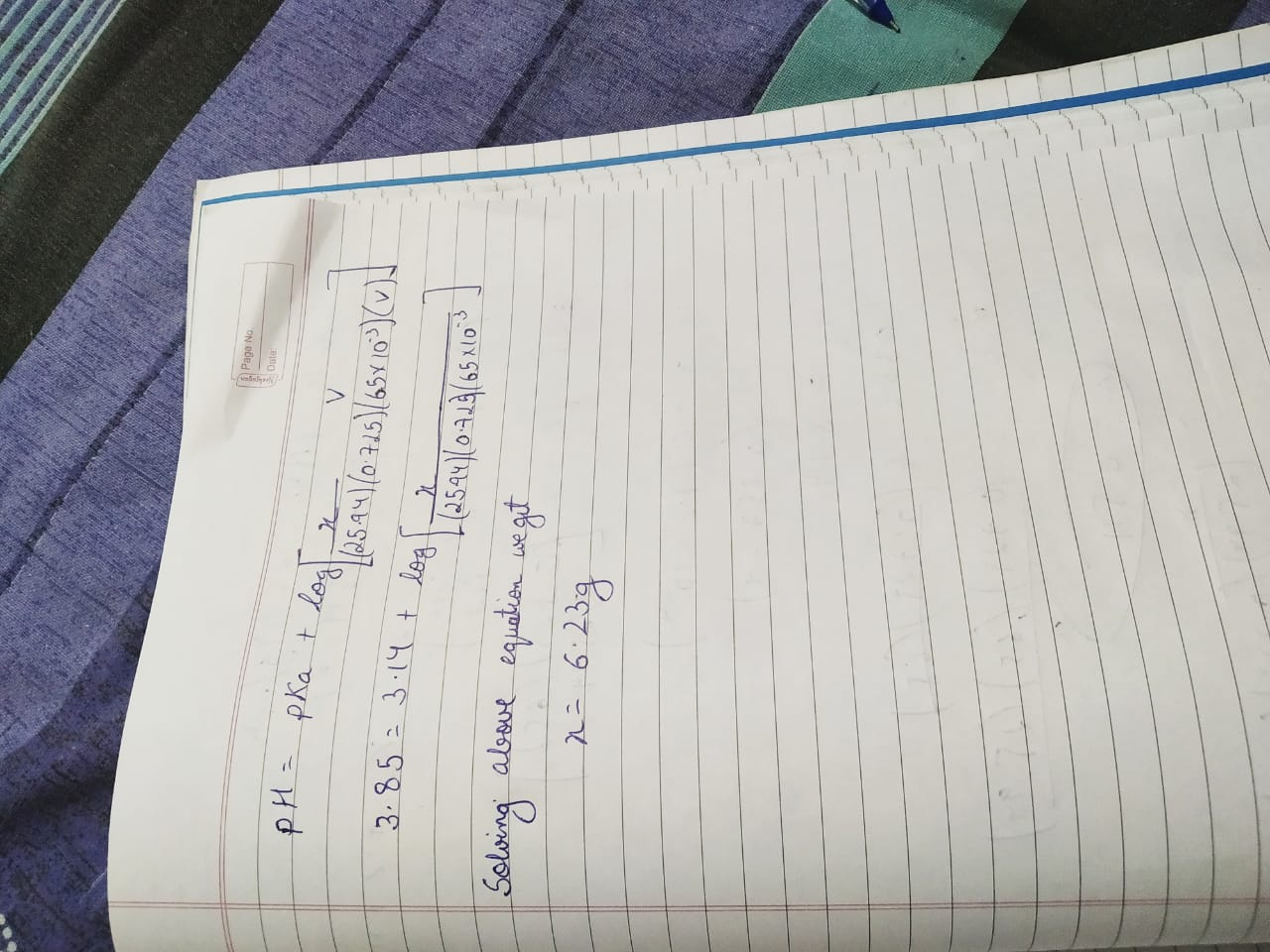#### Earn Coins

Coins can be redeemed for fabulous gifts.

Similar Homework Help Questions
• ### Consider a solution initially containing 0.300 mol of hydrogen fluoride (HF). How many grams of Naf (42.0 /mo vould...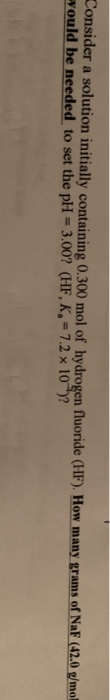Consider a solution initially containing 0.300 mol of hydrogen fluoride (HF). How many grams of Naf (42.0 /mo vould be needed to set the pH = 3.00? (HF, K, = 7.2 x 10")?

• ### How many grams of dipotassium succinate trihydrate (K2C4H4O4·3H2O, MW = 248.32 g/mol) must be added to...

How many grams of dipotassium succinate trihydrate (K2C4H4O4·3H2O, MW = 248.32 g/mol) must be added to 650.0 mL of a 0.0420 M succinic acid solution to produce a pH of 5.867? Succinic acid has pKa values of 4.207 (pKa1) and 5.636 (pKa2).

• ### How many grams of Na3PO4 (MW = 164 g/mol) are needed to make 282.3 mL of...

How many grams of Na3PO4 (MW = 164 g/mol) are needed to make 282.3 mL of solution that is 0.200 M sodium?

• ### How many grams (to the nearest 0.01 g) of NH4Cl (Mm = 53.49 g/mol) must be...

How many grams (to the nearest 0.01 g) of NH4Cl (Mm = 53.49 g/mol) must be added to 550. mL of 0.976-M solution of NH3 in order to prepare a pH = 9.15 buffer? What volume (to the nearest 0.1 mL) of 4.00-M NaOH must be added to 0.600 L of 0.300-M HNO2 to prepare a pH = 3.50 buffer?

• ### QUESTION 5                  8 MARKS a) How many grams of BaCl2.2H2O (MW = 244.27 g/mol) you need...

QUESTION 5                  8 MARKS a) How many grams of BaCl2.2H2O (MW = 244.27 g/mol) you need to prepare 250 mL of 0.15 M chloride ion solution? 2 marks b) It was established that this reagent was contaminated by CaCl2 (MW = 181.82 g). The content of calcium in the solid sample was found to be 4750 ppm (w/w). What was the percentage decrease in the molar Barium ion concentration caused by this contamination? 6 marks hi chemists I recieved this...

• ### How many grams (to the nearest 0.01 g) of NH4Cl (Mm = 53.49 g/mol) must be...

How many grams (to the nearest 0.01 g) of NH4Cl (Mm = 53.49 g/mol) must be added to 750. mL of 1.188-M solution of NH3 in order to prepare a pH = 9.90 buffer?

• ### How many grams (to the nearest 0.01 g) of NH4Cl (Mm = 53.49 g/mol) must be...

How many grams (to the nearest 0.01 g) of NH4Cl (Mm = 53.49 g/mol) must be added to 750. mL of 0.928-M solution of NH3 in order to prepare a pH = 8.95 buffer?

• ### How many grams of KCN (Mm = 65.1 g/mol) must be added to 900. mL of...

How many grams of KCN (Mm = 65.1 g/mol) must be added to 900. mL of 1.240-M solution of HCN in order to prepare a pH = 8.86 buffer?

• ### (3 pts) 4. You are asked to prepare a 0.100 M)glycine buffer pH 9.0. You are given a bottle of glycine (FW 75.1 g/mol) and a stock solution of 1.00 M NaOH. How many grams of glycine and how many...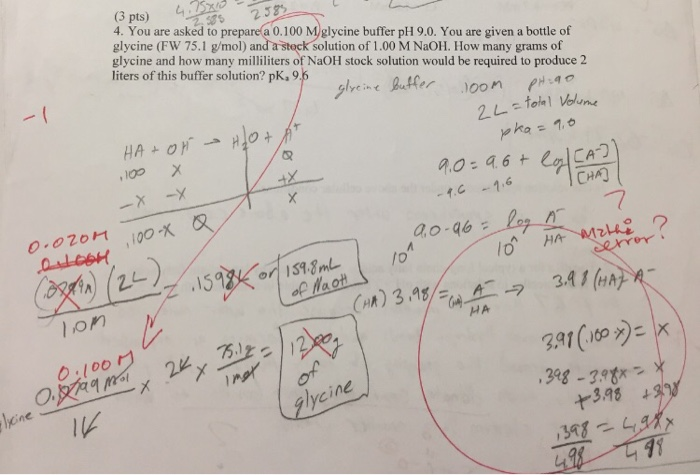(3 pts) 4. You are asked to prepare a 0.100 M)glycine buffer pH 9.0. You are given a bottle of glycine (FW 75.1 g/mol) and a stock solution of 1.00 M NaOH. How many grams of glycine and how many milliliters of NaOH stock solution would be required to produce 2 liters of this buffer solution? pk,95 alyeine tr oon 2 38 CHA) 7 3A1 (HA o.l0o glycine 3.98 (3 pts) 4. You are asked to prepare a 0.100 M)glycine...

• ### D Question 4 5 pts 2b) There are 0.10 moles of N2O4 and 0.20 moles of...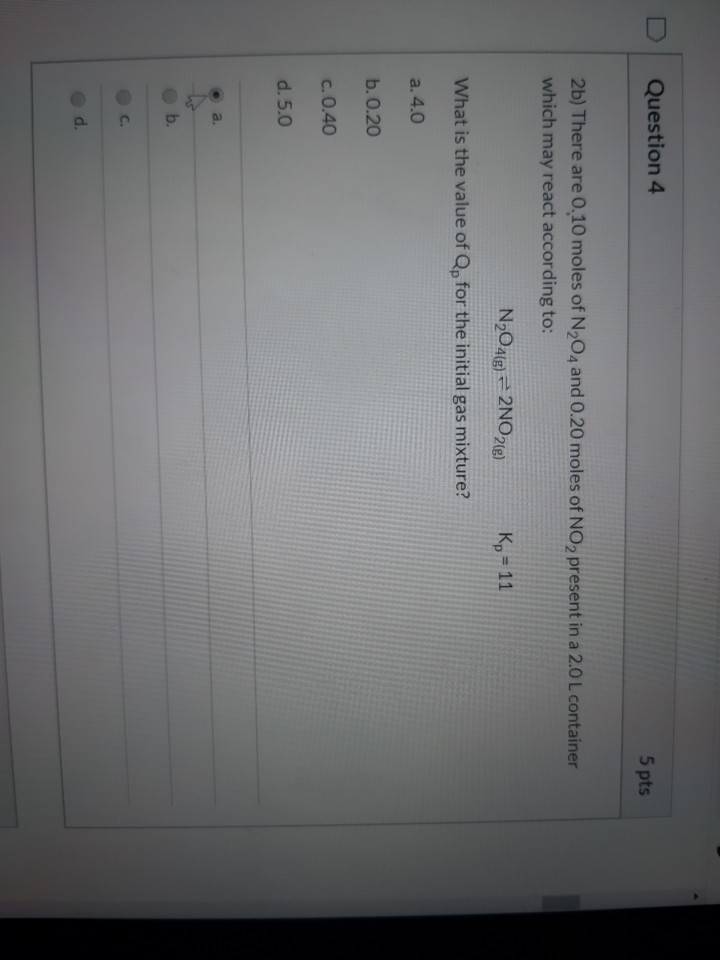D Question 4 5 pts 2b) There are 0.10 moles of N2O4 and 0.20 moles of NO 2 present in a 2.0 L container which may react according to: N2O4(g) = 2N028) Kp = 11 What is the value of Qp for the initial gas mixture? a. 4.0 b. 0.20 c. 0.40 d.5.0 . a. b. Consider the following equilibrium systems. Which direction will the reactions shift (reactants or products) when cooled? 200g + O2(g) = 2CO2(g) H2e+12(e)=2HI® H2(g) +126)...